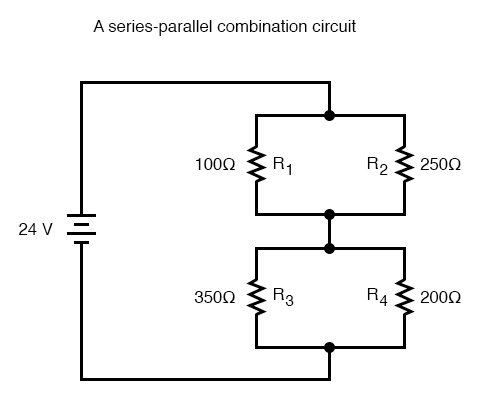Swati Talwar

HS-PS3-5

Combined series-parallel circuit meaning

Combined series-parallel circuit principle

Combined series-parallel circuit working

Combined series-parallel circuit calculations

# Combined Series-Parallel Circuits Study Guide

Series and parallel circuits combined examples show us the complexity of these circuits and how both the properties of series and parallel are in it.

# INTRODUCTION

A combined series-parallel circuit is a type of circuit connected in both series and parallel. The pathways are designed as the hardest electric series and parallel combination circuits. All the components of this circuit are connected end to end, forming a parallel flow of the circuit.

## PRINCIPLE OF COMBINED SERIES-PARALLEL CIRCUITS

• The current remains the same throughout the circuit.
• The sum of voltage all across the three resistors is equal to the other points' voltage.
• It follows the rule of thumb.

## WORKING OF COMBINED SERIES-PARALLEL CIRCUITS

We know that this circuit is neither a series nor a parallel, but it has the elements of both. This circuit has many paths for the current like in the parallel circuit, but there exists two sets of common points in the circuit. While solving the series-parallel combination circuit equation, you need to calculate the total resistance in both parts. To get a clear idea of how these circuits work, refer to the below given series and parallel circuit combined example.Source

## FORMULA OF COMBINED SERIES-PARALLEL CIRCUITS

• There is no definite formula for series and parallel circuit combination.
• So for calculating the average resistance of a series-parallel circui, we have to find the resistance by solving the resistance of parallel and series sections separately and combining them to find the right value of resistance.
• Further, to calculate the resonance frequency of the combined series-parallel circuit, we need to combine the formulas of both.

# SUMMARY

• The combination circuits are a type of mixed circuit that follows a unique path. Some flow through a single resistor path, while some flow in parallel through two or more points.
• The combination series-parallel circuit has equal voltage all across the circuit.
• Currents remain equal throughout the circuit.

## FAQs

1. How do you solve combined parallel and series circuits?

To solve these circuits, follow these instructions:

• See which resistors are used in the circuit
• Redraw the circuit until the circuit completes and gets reduced to the one equivalent resistor.
• Calculate current using Ohm's Law.
• Calculate the power dissipations.

2. What is the series-parallel combination?

It is a unique type of circuit that is connected in both series and parallel ways. The current remains the same throughout the circuit, and the sum of voltage all across the three resistors is equal to the other points' voltage.

3. What are the examples of series-parallel combinations?

Some examples are-

• Strong Christmas lights
• Light bulbs
• Multiplexer
• Wiring of car headlights
• Comparator

4. What is the formula for series-parallel combinations?

Parallel circuit:

1/Rt = 1/R1 1/R2 1/R3 ... Rt = R (t)total

Series circuit:

Total Resistance = R1 R2 R3……so on

5. What are the rules for series and parallel circuits?

• The combination circuit follows the rule of thumb.
• The outside current is the same throughout the circuit.
• The inside current is always less than the outside current.

We hope you enjoyed studying this lesson and learned something cool about Combined Series-Parallel Circuits! Join our Discord community to get any questions you may have answered and to engage with other students just like you! We promise, it makes studying much more fun!😎

1. Combination Circuits: https://www.physicsclassroom.com/class/circuits/Lesson-4/Combination-Circuits Accessed 19th April 2022.
2. Series-Parallel Circuits: https://www.oakton.edu/user/1/agero/elt101/presentations/Chapter06.pdf Accessed 19th April 2022.
3. Direct Current: https://www.allaboutcircuits.com/textbook/direct-current/chpt-7/analysis-technique/ Accessed 19th April 2022.
4. What is a Series-Parallel Circuit? https://www.allaboutcircuits.com/textbook/direct-current/chpt-7/what-is-a-series-parallel-circuit/ Accessed 19th April 2022.